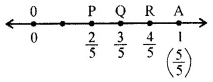ML Aggarwal Class 6 Solutions Chapter 6 Fractions Ex 6.2 for ICSE Understanding Mathematics acts as the best resource during your learning and helps you score well in your exams.

## ML Aggarwal Class 6 Solutions for ICSE Maths Chapter 6 Fractions Ex 6.2

Question 1.
Show the fractions $$\frac{2}{5}, \frac{3}{5}, \frac{4}{5} \text { and } \frac{5}{5}$$ on a number line.
Solution:Question 2.
Show $$\frac{1}{8}, \frac{2}{8}, \frac{3}{8} \text { and } \frac{7}{8}$$ on a number line.
Solution:Question 3.
Show $$\frac{0}{10}, \frac{1}{10}, \frac{3}{10}, \frac{5}{10}, \frac{7}{10} \text { and } \frac{10}{10}$$ on a number line.
Solution: